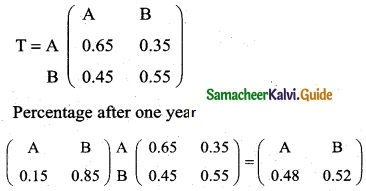Tamilnadu State Board New Syllabus Samacheer Kalvi 12th Business Maths Guide Pdf Chapter 1 Applications of Matrices and Determinants Ex 1.3 Text Book Back Questions and Answers, Notes.

## Tamilnadu Samacheer Kalvi 12th Business Maths Solutions Chapter 1 Applications of Matrices and Determinants Ex 1.3

Question 1.
The subscription of a magazine sends out a letter to a large mailing list inviting subscriptions for the magazine. Some of the people receiving this letter already subscribe to the magazine while others do not. From this mailing list, 45% of those who already subscribe will subscribe again while 30% of those who do not now subscribe will subscribe. On the last letter, it was found that 40% of those receiving it ordered a subscription. What percent of those receiving the current letter can be expected to order a subscription?
Solution:
Transition Probability MatrixS = 36%; F = 64%
∴ 36% of those receiving the current letter can be expected to order a subscriptionQuestion 2.
Anew transit system has just gone into operation in Chennai. Of those who use the transit system this year, 30% will switch over to using metro train next year and 70% will continue to use he transit system. Of those who use metro train this year and 70% will continue to use metro train next year and 30% will switch over to transit system. Suppose the population of Chennai city remains constant and that 60% of the commuters use the transit system and 40% of the commuters use metro train this year.
(i) What percent of commuters will be using the transit system after one year?
(ii) What percent of commuters will be using the transit system in the one run?
Solution:
Transition Probability MatrixS = 54%; C = 46%
Equilibrium will be reached in the long run
At equilibrium we must have
(S C) T = (S C) where S + C = 1
(S C) = $$\left(\begin{array}{ll} 0.7 & 0.3 \\ 0.3 & 0.7 \end{array}\right)$$ = (S C)
0.7S + 0.3C = S
0.7S + 0.3 (1 – S) = S
0.7S + 0.3 – 0.3S = S
0.4S + 0.3 = S
0.3 = S – 0.4S ⇒ 0.6S = 0.3
S = $$\frac { 0.3 }{ 0.6 }$$ = 0.50
∴ 50% of the commuters will be transit system the long run.Question 3.
Two types of soaps A and B are in the market. Their present market shares are 15% for A and 85% for B. Of those who bought A the previous year, 65% contionues to buy it again while 35% switch over to B. Of those who bought B the previous year, 55% buy it again and 45% switch over to A. Find their market shares after one year and when is the equilibrium reached?
Solution:
Transition Probability MatrixEquilibrium will be reached in the long run
At equilibrium we must have
(A B) T = (A, B) where A + B = 1
(A B) = $$\left(\begin{array}{ll} 0.65 & 0.35 \\ 0.45 & 0.55 \end{array}\right)$$ = (A B)
0.65 A + 0.45 B = A
0.65 A + 0.45 (1 – A) = A
0.65 A + 0.45 – 0.45 A = A
0.20 A + 0.45 = A
A – 0.20 A = 0.45
0.80 A = 0.45
A = $$\frac { 0.45 }{ 0.80 }$$ = 0.5625 and B = 04375
In the long sum {∴ B = 1 – A}
∴ A = 56.25 % and B = 43.75%Question 4.
Two products A and B currently share the market with shares of 50% and 50% each respectively. Each week some brand switching takes place. Of those who bought A the previous week, 60% buy it again whereas 40% switch over to B. Of those who bought B the previous week, 80% buy it again whereas 20% switch over to A. Find their shares after one week and after two weeks. If the price war continues, when is the equilibrium reached?
Solution:
Transition Probability MatrixEquilibrium will be reached in the long run.
At equilibrium, we must have
(A B) T = (A B) where A + B = 1
(A B) = $$\left(\begin{array}{ll} 0.60 & 0.40 \\ 0.20 & 0.80 \end{array}\right)$$ = (A B)
0.60 A + 0.20 B = A
0.60 A+ 0.20 (1 – A) = A
0.60 A + 0.20 – 0.20 A = A
0.40 A + 0.20 = A
0.20 = A – 0.40 A
0.60 A = 0.20
A = $$\frac { 0.20 }{ 0.60 }$$ = 0.33 and B = 0.67
{∴ B = 1 – A}
In the long run
∴ A = 33% and B = 67%